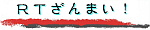\$B8=:_\$N>l=j(B :
 \$B8!:w%Z!<%8(B
English
\$B8!:w

# \$B8=:_\$N8!:w<0(B

• (70)
• \$B8!:w\$9\$kG/(B: 2001.
• \$BI=<(=g(B: \$B%Z!<%88x3+F|(B.
• \$B5-;v\$NoJ8=q(B, \$B%j%=!<%9(BDB, \$B\$*

# \$B8!:w7k2L(B

• \$B%P!<%_%s%,%`!"%V%j%s%I%j!<%W%l%\$%9IU6a\$N1?2O(B
[\$B\$* (\$B%Z!<%88x3+(B 2001. 12. 8, \$B:G=*99?7(B 2002. 10. 5)
• \$B%f%K%*%s%8%c%C%/\$NH>4z(B
[\$B\$* (\$B%Z!<%88x3+(B 2001. 9. 21, \$B:G=*99?7(B 2003. 5. 29)
• \$B%P!<%_%s%,%`>pJs%Z!<%8(B
\$BEv%5%\$%H\$N\$"\$A\$3\$A\$K\$"\$k!"(B \$B%P!<%_%s%,%`4XO"\$N>pJs\$r=8\$a\$F\$_\$^\$7\$?!#(B
(\$B%Z!<%88x3+(B 2001. 8. 27, \$B:G=*99?7(B 2003. 5. 22)

• \$B2hA|\$KCx:n8"I=<(\$NJ8;zNs\$,\$G\$k>l9g!"(B \$B\$3\$A\$i\$r%/%j%C%/!#(B
\$B9bLZ(B \$BN<(B webmaster@takagi-ryo.ac
(c) R. Takagi 2002 - 2021. All rights reserved.